Question

For the Op-Amp in the figure below find the magnityde of the output voitage Vout R....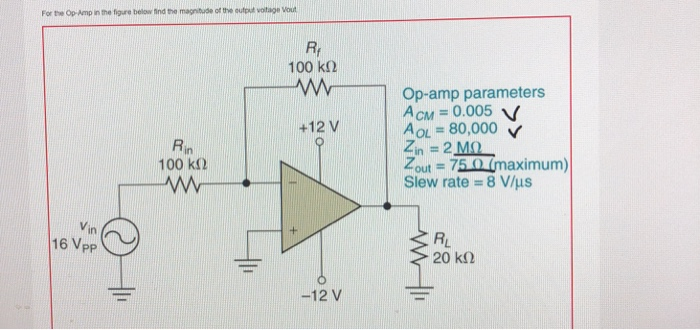For the Op-Amp in the figure below find the magnityde of the output voitage Vout R. 100 kΩ Op-amp parameters +12 V AOL = 80,000 22 Mo Tout 75 0(maximum) Slew rate-8 V/μs Rin 100 kΩ in 16 Vpp RL -12 V

One of the properties of an ideal op amp is that it can drive any load. In other words, the ideal op amp will supply whatever output current is necessary to achieve the output voltage set by the input and the feedback network.

Consider the circuit with and without a load resistor. Without a load resistor (i.e. RL→∞) there is clearly only one path for the output current to flow -- into the feedback resistor, and this current also flows entirely into the input resistor since no current flows into an ideal op amp's inputs. This sets the relationship between the input and output voltages for the inverting amplifier. Adding a finite load resistance doesn't affect the feedback network nor the relationship between input and output -- it just means that the op amp needs to supply more output current (the usual current into the feedback network, as well as the current into the load resistor to satisfy Ohm's Law). Since an ideal op amp can output any current necessary, the load resistor does not affect the transfer function.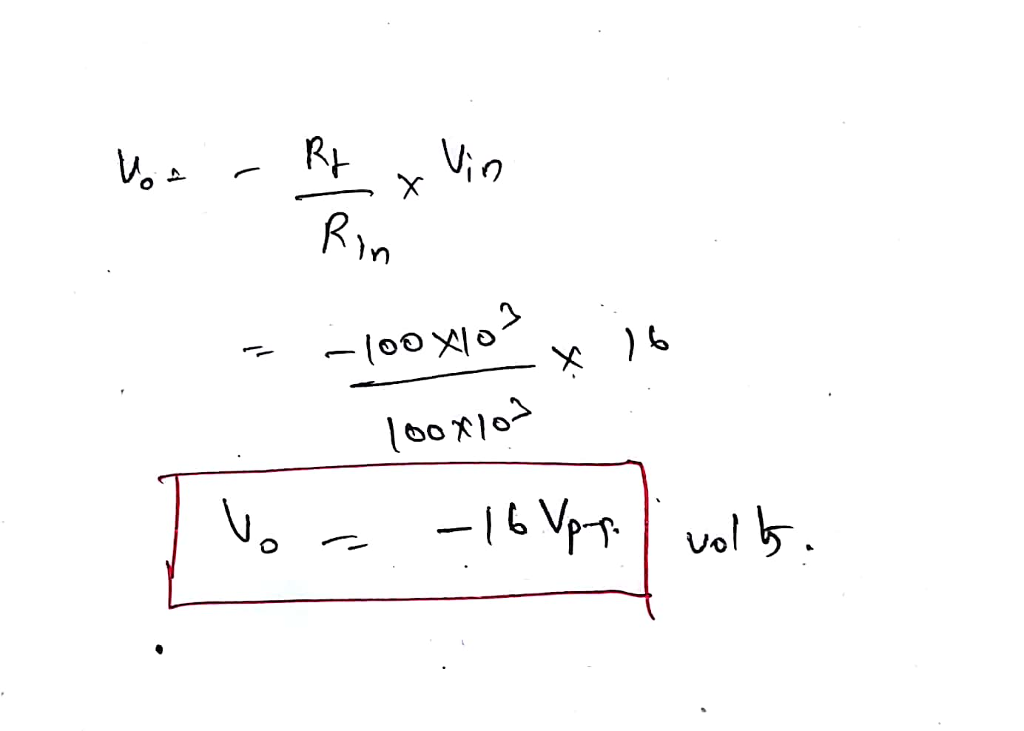Earn Coins

Coins can be redeemed for fabulous gifts.

Similar Homework Help Questions
• A. (10 pts) Implement the voltage amplifier shown below using an ideal op amp circuit. You have o...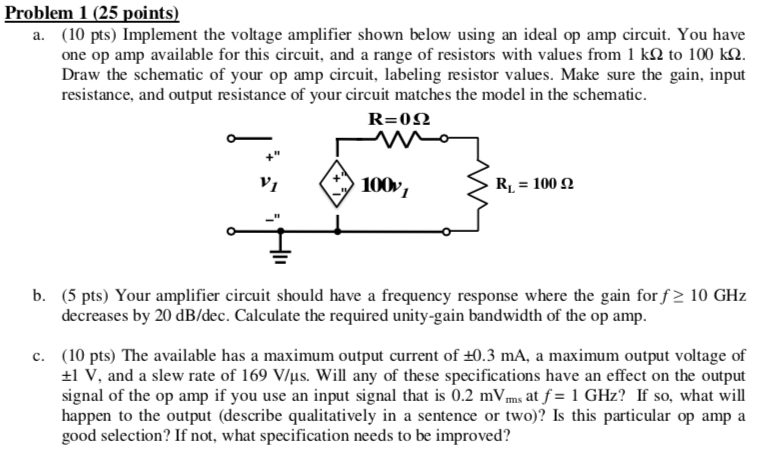a. (10 pts) Implement the voltage amplifier shown below using an ideal op amp circuit. You have one op amp available for this circuit, and a range of resistors with values from 1 kΩ to 100 ka. Draw the schematic of your op amp circuit, labeling resistor values. Make sure the gain, input resistance, and output resistance of your circuit matches the model in the schematic. R=012 *100v, RL 100 b. (5 pts) Your amplifier circuit should have a frequency...

• A non-inverting amplifier using OP-amp is given in Figure Q4c. (8) Vout Vin 99 kN RL...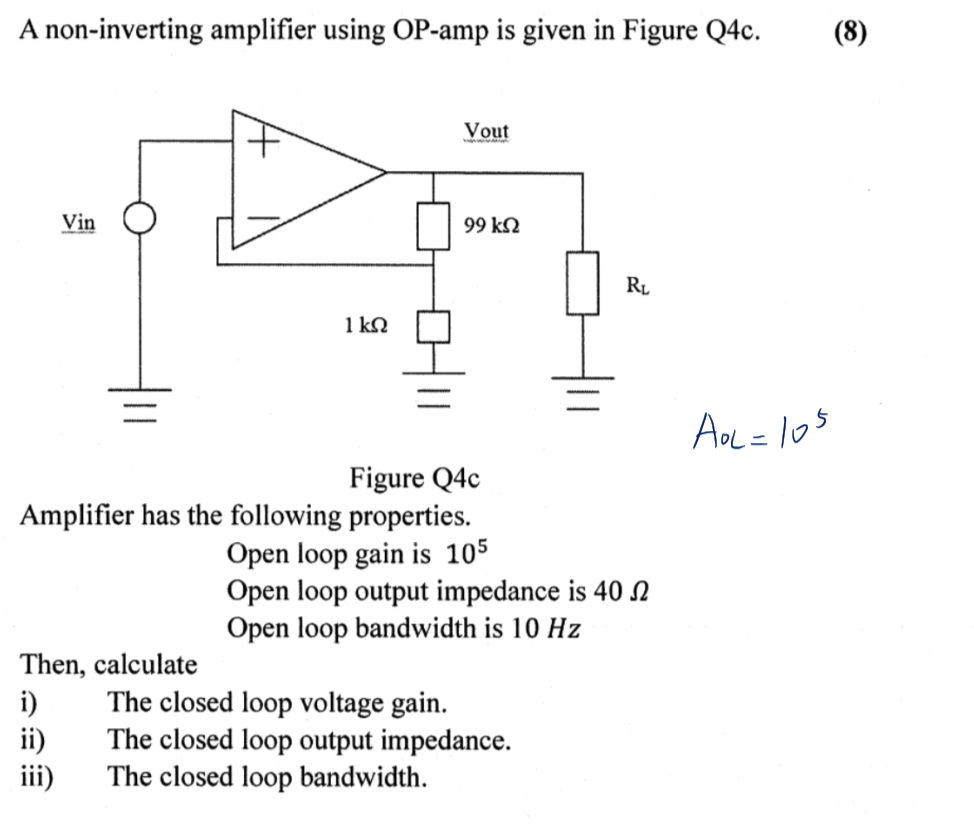A non-inverting amplifier using OP-amp is given in Figure Q4c. (8) Vout Vin 99 kN RL 1 kQ AoL = 10s Figure Q4c Amplifier has the following properties. Open loop gain is 105 Open loop output impedance is 40 N Open loop bandwidth is 10 Hz Then, calculate The closed loop voltage gain. The closed loop output impedance. The closed loop bandwidth. i) ii) iii)

• Just need answers Question 26 It takes an op-amp 22 μs to change its output from...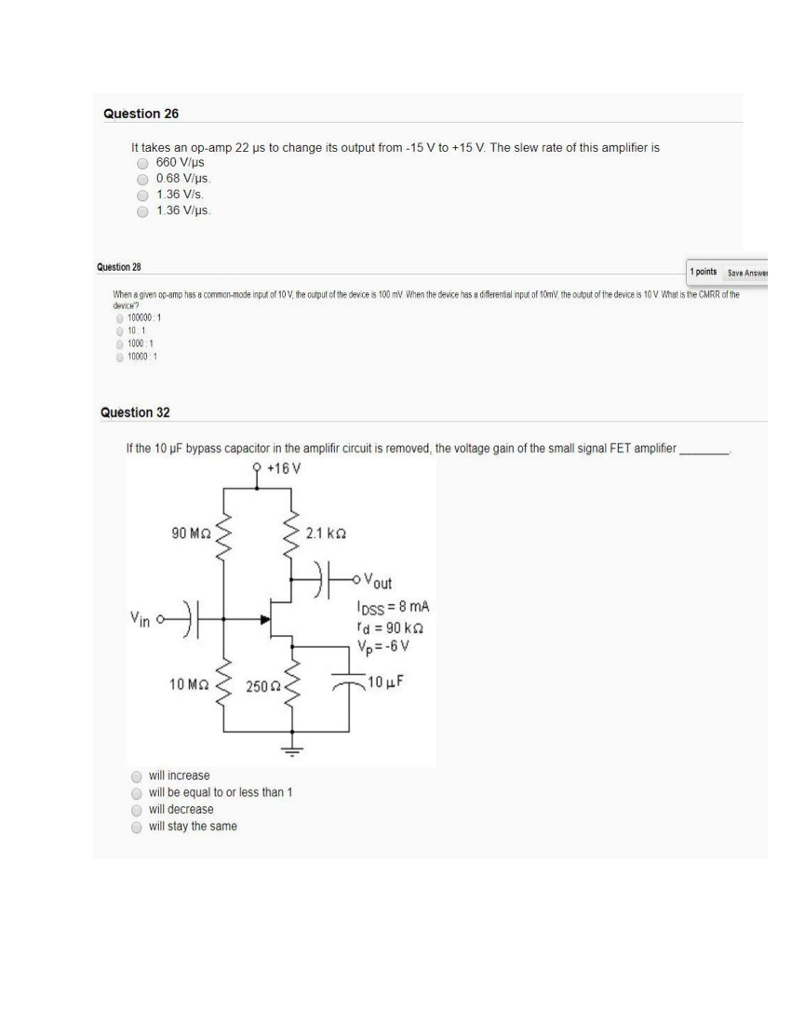Just need answers Question 26 It takes an op-amp 22 μs to change its output from -15 V to +15 V. The slew rate of this amplifier is 660 V/ps O 0.68 Vlus O 1.36 Vis 1.36 V/us Question 28 1 points 5ave Answe the ag en opamo has 8 como mode devCH"? p녀 of 10V,the outut cfthe devce s 100 ㎷When the de ce has a diferental mplof10mV theo tutofthe deces1 VWha'sneCMRR ofthe 100000 1 0 10 1 1000:1...

• Q 1. An op amp with an open-loop gain of 6 x 105 and Vcc =...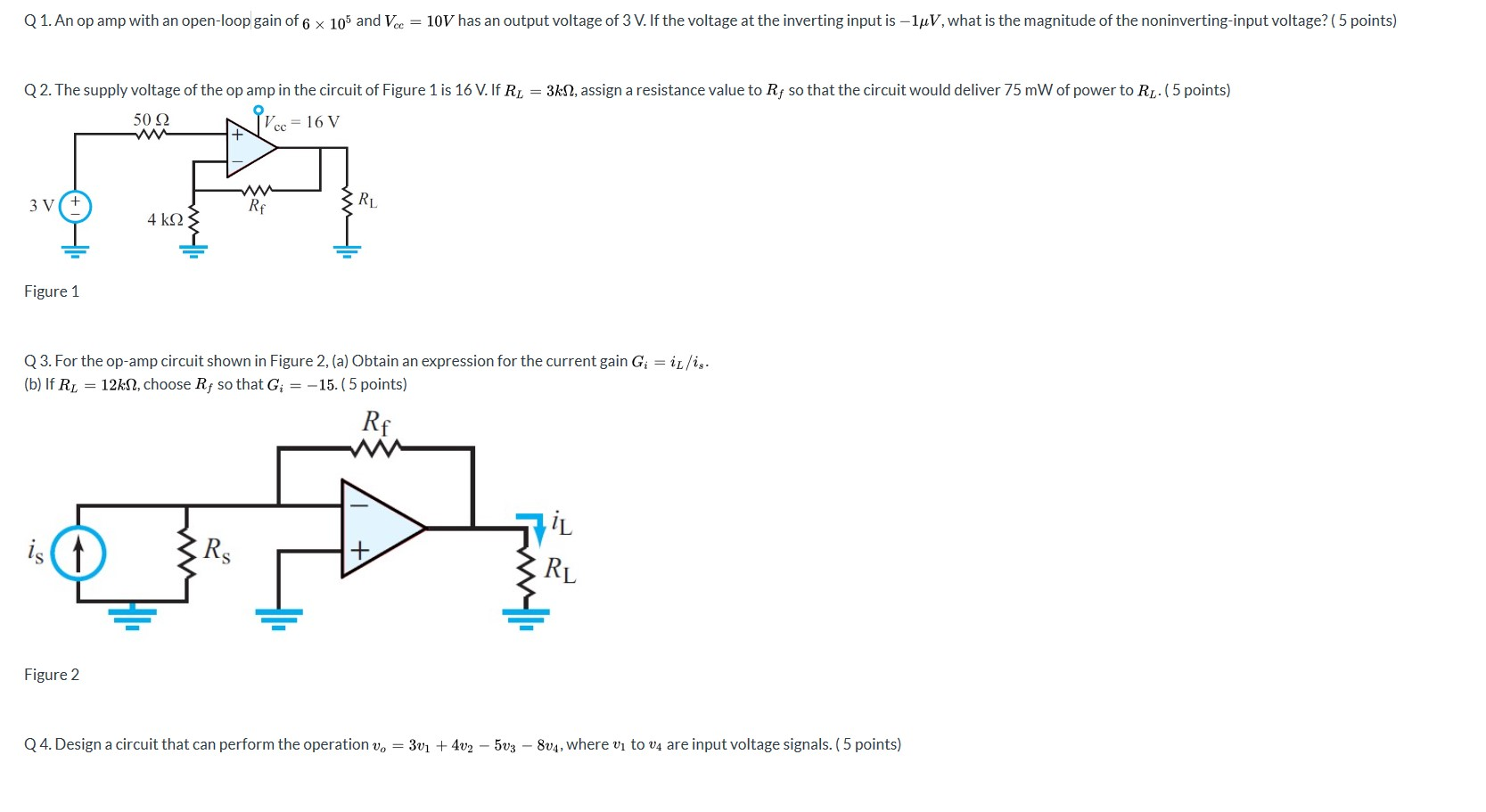Q 1. An op amp with an open-loop gain of 6 x 105 and Vcc = 10V has an output voltage of 3 V. If the voltage at the inverting input is -luV, what is the magnitude of the noninverting-input voltage? (5 points) Q2. The supply voltage of the op amp in the circuit of Figure 1 is 16 V. If R = 3kN, assign a resistance value to Rf so that the circuit would deliver 75 mW of power...

• having a de is to cascade Op amps wi Write an Figure P2.42 243. The objective...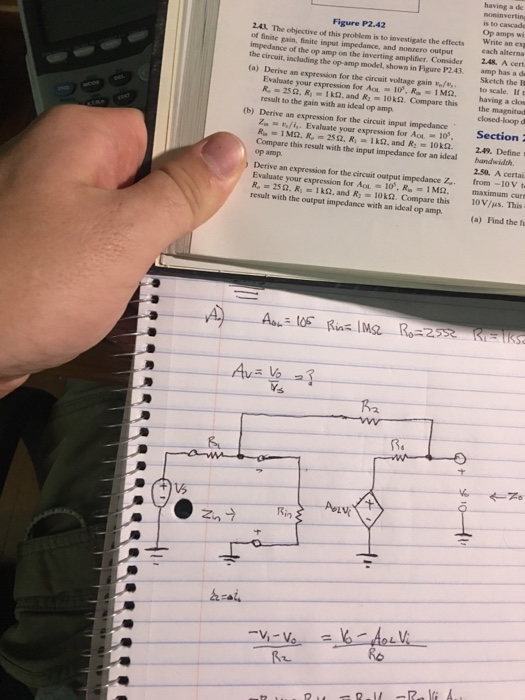having a de is to cascade Op amps wi Write an Figure P2.42 243. The objective of this probiem is to investigate the effects of finite gain, finite input impedance, and nonzero output impedance of the op amp on the inverting amplifier. Consider the effects ex 248. A cert the circui, including the op-amp model, shown in Figure P2.43. amp has a d (a) Derive an expression for the circuit voltage gain o/to scale. If t Sketch the E Evaluate...

• The op amp in the circuit in (Figure 1) is ideal. Suppose R-16 kΩ Part A...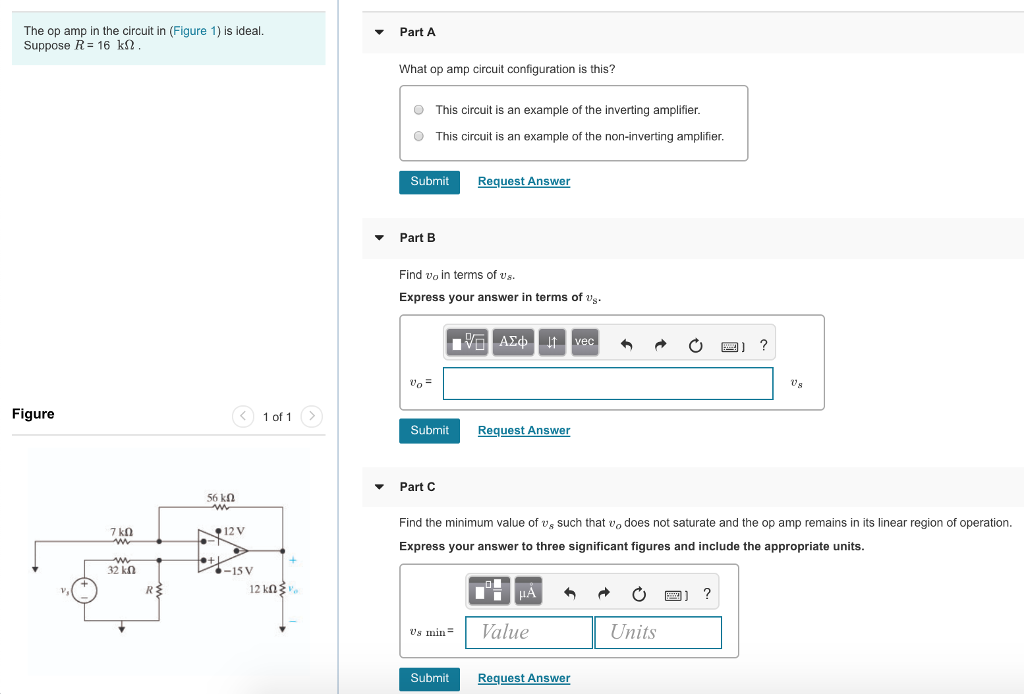The op amp in the circuit in (Figure 1) is ideal. Suppose R-16 kΩ Part A What op amp circuit configuration is this? O This circuit is an example of the inverting amplifier o This circuit is an example of the non-inverting amplifier Submit Request Answer ▼ Part B Find vo in terms of vs Express your answer in terms of vs 07 Figure 1 of 1 > Submit Request Answer Part C 56 kΩ Find the minimum value of...

• Problem 2: Assume ideal op amp in Figure 2 below. (a). Find Vo, when va =...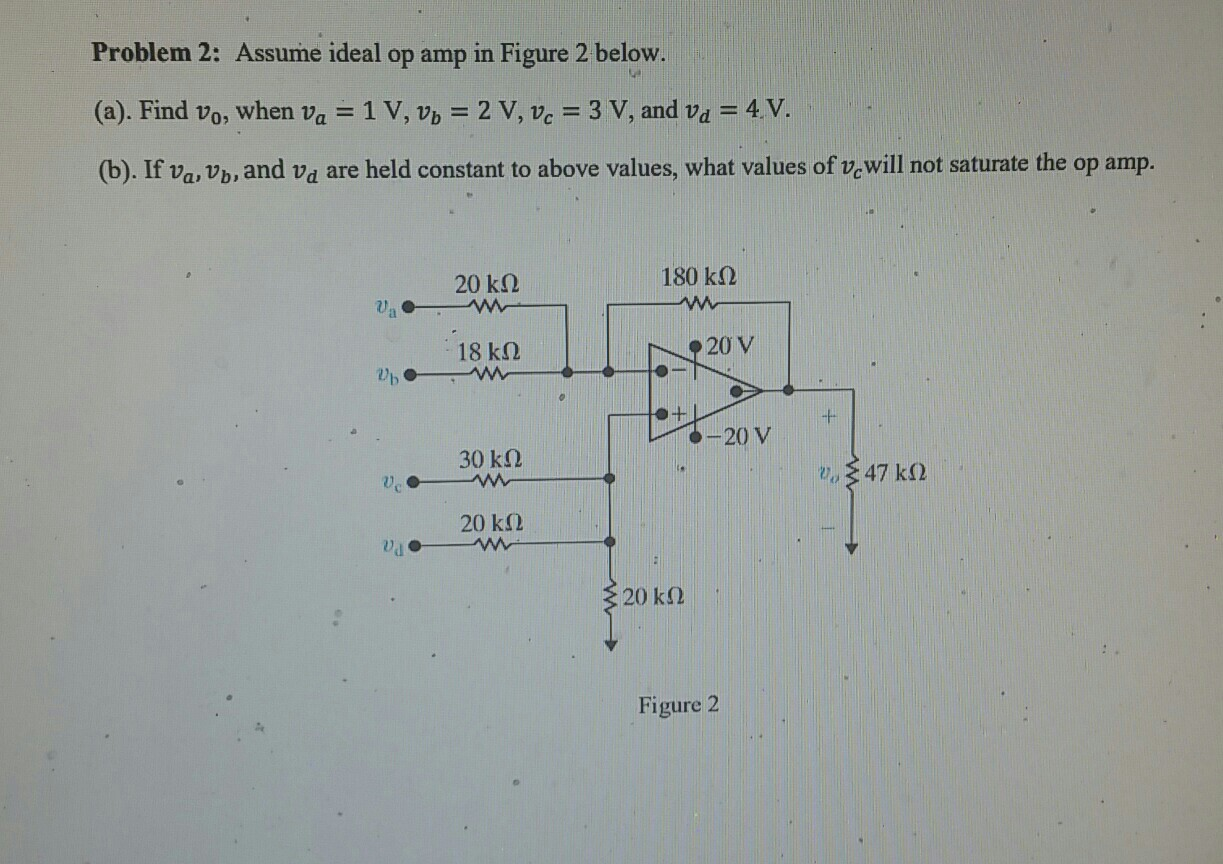Problem 2: Assume ideal op amp in Figure 2 below. (a). Find Vo, when va = 1 V, v,-2 V, vc = 3 V, and v.-4-V. (b). If va, Vb, and va are held constant to above values, what values of vewill not saturate the op amp. 20 kΩ 180 kΩ 18 kΩ 20 V -t "20 V 30 kΩ 20 kΩ 20 kΩ Figure 2

• Aufilo polasi For the op amp shown below, the output saturation voltages of the op amp...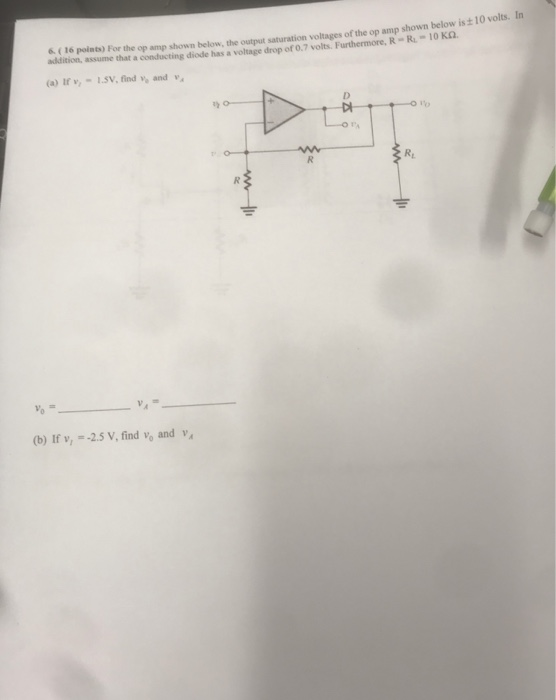Aufilo polasi For the op amp shown below, the output saturation voltages of the op amp shown below is t 10 volts. In addition, assume that conducting 6. ( 16 points) the a conducting diode has a voltage drop of 0.7 volts. Furthermore, R- R-10 KQ (a) Ir v1.SV, find and v (b) If v, =-2.5 V, find Vo and va

• for the followingng op-amp show analattically the effect of the variation of R on the output...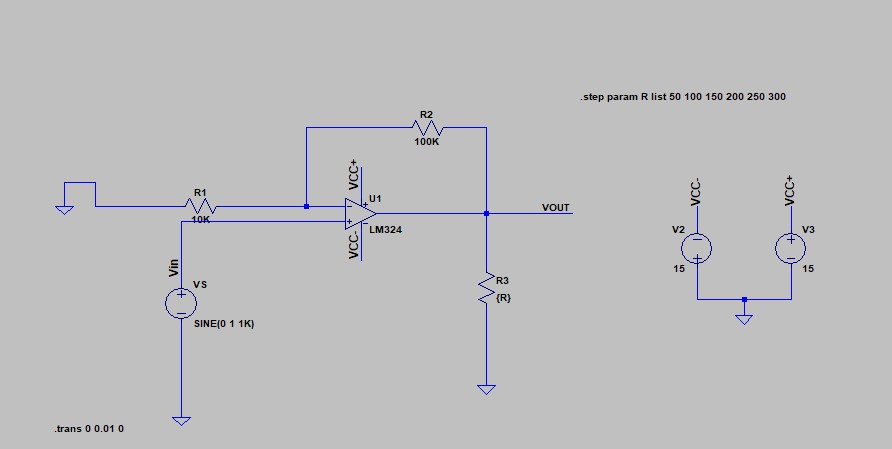for the followingng op-amp show analattically the effect of the variation of R on the output votlage step param R list 50 100 150 200 250 300 R2 100K / VCC+ VCC- VCC+ -VCC+ VV VOUT + VCC- {R} SINE(O 1 1K) Y trans 0 0.01 0

• For the op amp circuit in the given figure, suppose vs 24u(t) V. Find v(t) for...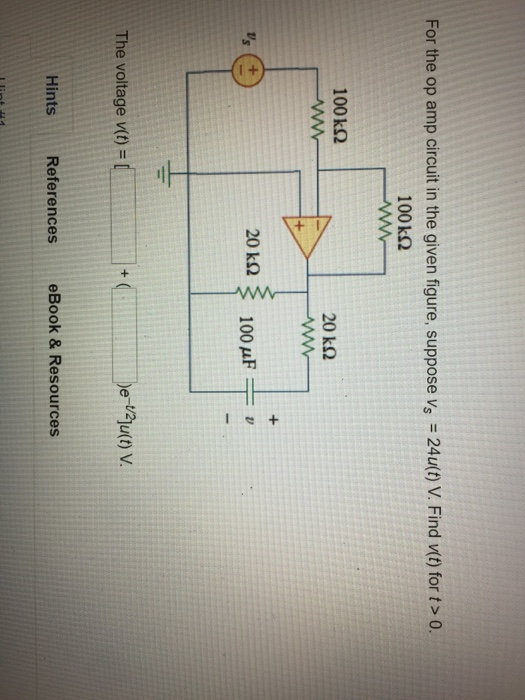For the op amp circuit in the given figure, suppose vs 24u(t) V. Find v(t) for t> 0. 100 kΩ 100 kΩ The voltage v(t) = Hints References eBook & Resources